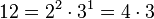# Classification of groups of order 12

This article gives specific information, namely, classification, about a family of groups, namely: groups of order 12.
View classification of group families | View classification of groups of a particular order |View other specific information about groups of order 12

## Statement

There are, up to isomorphism of groups, five groups of order 12, namely:

Group Second part of GAP ID (GAP ID is (12,second part)) Abelian? 2-Sylow subgroup Is the 2-Sylow subgroup normal? Is the 3-Sylow subgroup normal?
dicyclic group:Dic12 1 No cyclic group:Z4 No Yes
cyclic group:Z12 2 Yes cyclic group:Z4 Yes Yes
alternating group:A4 3 No Klein four-group Yes No
dihedral group:D12 4 No Klein four-group No Yes
direct product of Z6 and Z2 5 Yes Klein four-group Yes Yes

## Facts used

1. Order is product of Mersenne prime and one more implies normal Sylow subgroup

## Proof

### Preliminary observation on Sylow subgroups

Note that the order is:$\! 12 = 2^2 \cdot 3^1 = 4 \cdot 3$

The 2-Sylow subgroup is thus one of the groups of order 4 (either cyclic group:Z4 or Klein four-group) an the 3-Sylow subgroup is the unique group of order 3, namely cyclic group:Z3.

### Either the 2-Sylow subgroup or the 3-Sylow subgroup is normal

This follows from Fact (1).

### Classification in case of both Sylow subgroups being normal

There's a total of two groups of this type: cyclic group:Z12 and direct product of Z6 and Z2.

In this case, by equivalence of definitions of finite nilpotent group, the whole group is a nilpotent group and occurs as an internal direct product of its 2-Sylow subgroup and 3-Sylow subgroup. The 2-Sylow subgroup is one of the two possible groups of order 4 (i.e., cyclic group:Z4 and Klein four-group) and the 3-Sylow subgroup is cyclic group:Z3. There are thus$2 \times 1 = 2$ possibilities:

Some other related facts:

### Classification in case the 2-Sylow subgroup is normal and the 3-Sylow subgroup is not normal

There is a total of one group of this type: alternating group:A4.

In this case, the whole group is an internal semidirect product of its 2-Sylow subgroup (which is normal) and its 3-Sylow subgroup, isomorphic to cyclic group:Z3, which is not normal. We are assuming a nontrivial semidirect product, hence, because the acting group is cyclic of prime order, it must be a faithful semidirect product.

The case that the 2-Sylow subgroup is cyclic group:Z4 does not arise because the automorphism group of cyclic group:Z4 is cyclic group:Z2, which has no subgroup of order three.

The case that the 2-Sylow subgroup is Klein four-group does give rise to a unique possibility: the automorphism group is symmetric group:S3, and the acting cyclic group:Z3 acts via the embedding A3 in S3. We thus get a semidirect product of a Klein four-group and a cyclic group of order three where a generator of the latter acts by a 3-cycle on the non-identity elements. The group obtained this way is alternating group:A4.

### Classification in case the 3-Sylow subgroup is normal and the 2-Sylow subgroup is not normal

In this case, the whole group is an internal semidirect product of its normal 3-Sylow subgroup cyclic group:Z3, and its 2-Sylow subgroup. To specify the internal semidirect product is equivalent to specifying a homomorphism from the 2-Sylow subgroup to the automorphism group of cyclic group:Z3.

The automorphism group of the 3-Sylow subgroup (cyclic group:Z3) is isomorphic to cyclic group:Z2, with the non-identity automorphism being the inverse map. Thus, specifying the internal semidirect product is equivalent to specifying a homomorphism from the 2-Sylow subgroup to cyclic group:Z2. Since we're assuming a nontrivial semidirect product, the homomorphism must be nontrivial. We consider two cases:

• The 2-Sylow subgroup is cyclic group:Z4: In this case, the only nontrivial homomorphism is the surjective homomorphism that reduces an element (originally mod 4) to the element mod 2. The group we get as semidirect product in this fashion is dicyclic group:Dic12.
• The 2-Sylow subgroup is Klein four-group: In this case, there are three equivalent (up to automorphisms of the Klein four-group) nontrivial homomorphisms, with kernels the various possibilities for Z2 in V4. They all give answers that are isomorphic as groups, namely dihedral group:D12 (which is also expressible as the direct product of symmetric group:S3 and cyclic group:Z2).